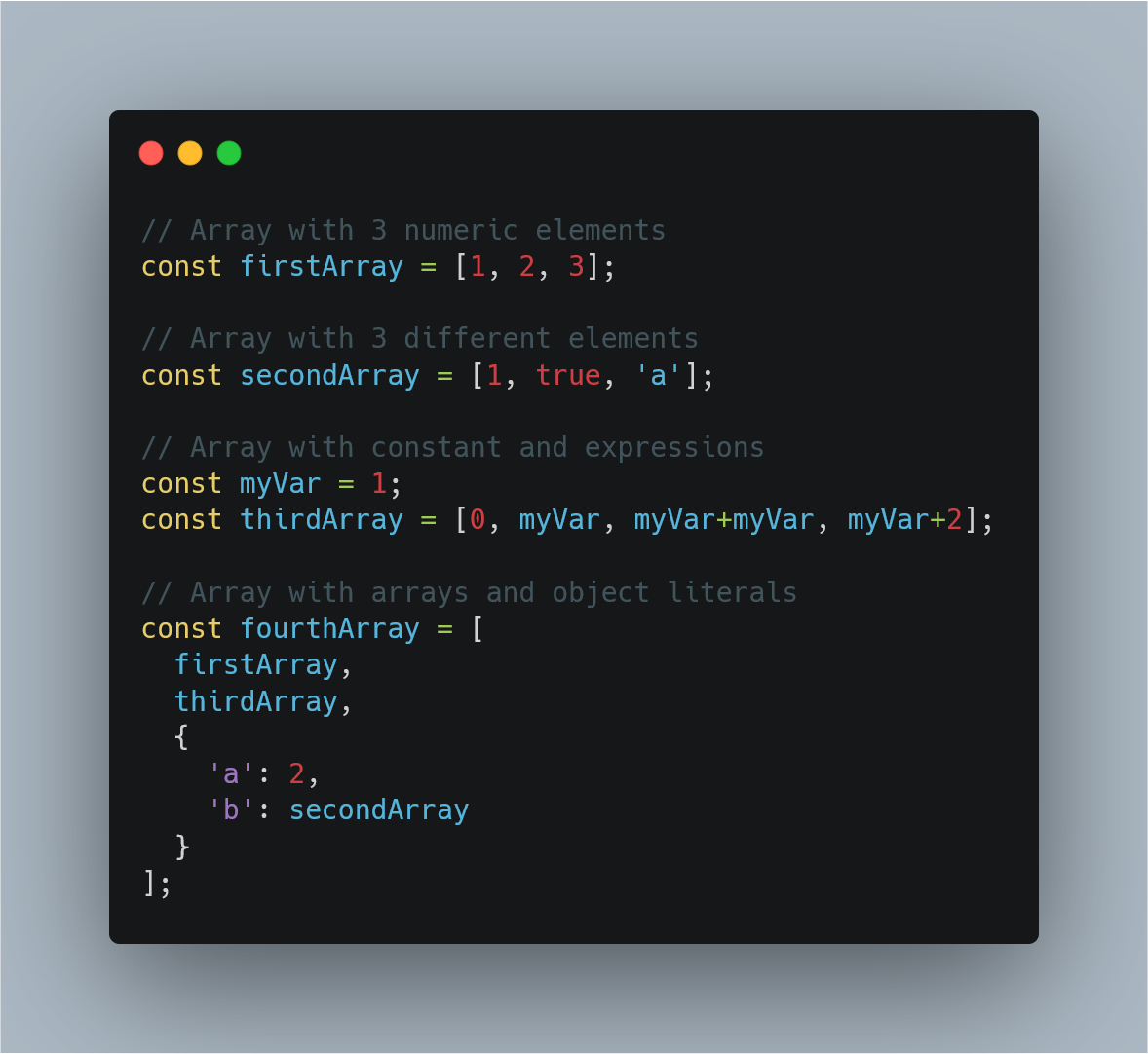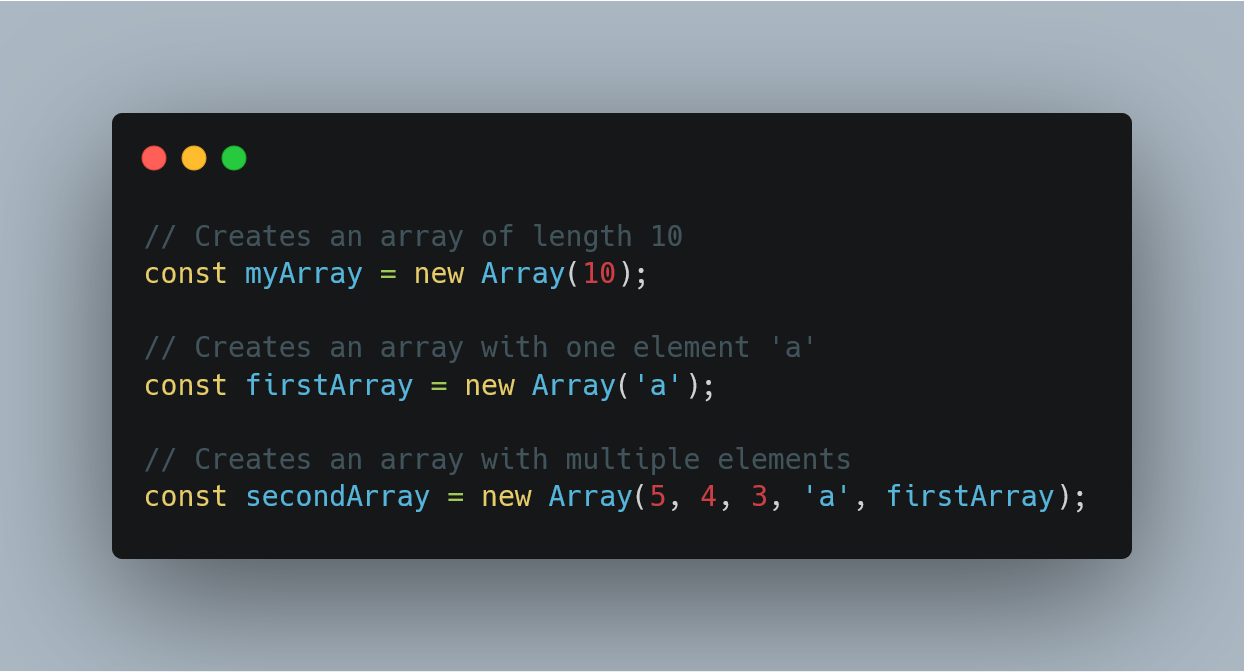1580527250

# JavaScript Array.flatMap()

Now lets up the game and introduce “flatMap”. It combines the steps of first mapping over the array with `map()` & then calling `flat()`. Instead of calling 2 methods, just use `flatMap()` 👍

``````const foods = ['🍫', '🍦'];

// ❌ map + flat
foods.map(food => [food, '😋']).flat();

// ✅ flatMap
foods.flatMap(food => [food, '😋']);

// Result
// ['🍫', '😋', '🍦', '😋']

``````

## How `flatMap()` works?

Let’s go through step-by-step what `flatMap()` is doing. I was a bit confused when I first learned this one. Cause I thought it flattens and then it does the mapping. But no 🙅. It first `map()` and then it `flat()`.

``````const names = ['jane', 'john'];

// Step 1: map
const nestedArray = names.map((name, index) => [name, index]);
// [ ['jane', 1], ['john', 2 ] ]

``````

So now we have a nested array. And we can use `flat()` to flatten the array.

``````const nestedArray = [
['jane', 1],
['john', 2]
];

nestedArray.flat();
// [ 'jane', 1, 'john', 2 ]

``````

Of course, we can shorten this and just call `flatMap()`. Let’s take a look 👀

``````const names = ['jane', 'john'];

const result = names.flatMap((name, index) => [name, index]);

// [ 'jane', 1, 'john', 2 ]

``````

And Voila! We have the same result 👍

## `flatMap` only flattens 1-level deep

With `flat()`, it accepts a parameter where you set the depth. What this means is you can specify how deep a nested array should be flattened.

``````const depth1 = [, ];
depth1.flat(); // same as depth.flat(1)
// [1, 2]

const depth2 = [[[1, 2]]];
depth2.flat(2);
// [1, 2]

``````

Now for `flatMap()`, you can only go 1-level deep.

``````const names = ['jane'];

names.flatMap((name, index) => [[name, index]]);
//  [ ['jane', 1] ]

``````

Let’s break this into 2 steps, so you can see what’s going on.

``````const names = ['jane'];

// Step 1: created a 2-level deep array
const twoLevelDeep = names.map((name, index) => [[name, index]]);
// [ [ ['jane', 1] ] ]

// Step 2: flat using depth 1
twoLevelDeep.flat();
//  [ ['jane', 1] ]

``````

But if you do it separately, I can pass a `depth` parameter and flatten it completely:

``````twoLevelDeep.flat(2);
// [ 'jane', 0, 'john', 1 ]

``````

So, if you want it to flatten beyond depth of 1. Then it is better to NOT use `flatMap()` and just call the methods separately 👍

## `flatMap` to filter item

One really cool you can do with `flatMap` is to remove an element. In this example, I want to remove all negative numbers.

``````const numbers = [1, 2, -3, -4, 5];

numbers.flatMap(number => {
return number < 0 ? [] : [number];
});

// [ 1, 2, 5]

``````

That’s really cool! It’s like acting like a `filter`. But how is this actually working. The secret is the empty array. Let’s see what I mean.

``````const emptyNestedArray = [[], 1];

emptyNestedArray.flat();
// [ 1 ]

``````

When you try to flatten an element that’s an empty array, it simply removes that item. So we can use that knowledge to make `flatMap` act kind of like `filter` method. Neat right! 👍

## Resources

Originally published at https://www.samanthaming.com

#javascript #array #web-development

## Buddha Community1599722400

## Javascript Array FlatMap Example | JS Array flatMap()

Javascript Array flatMap() is an inbuilt method that maps each element using the map function, then flattens the result into the new array. It is identical to the Javascript Array  map followed by the Javascript Array  flat of depth 1, but the flatMap() method is often quite useful, as merging both into one method is slightly more efficient.

### Javascript Array FlatMap Example

The array.flatMap() is an inbuilt Javascript function which is used to flatten the input array element into the new array. Array flatMap() first of all map every element with the help of mapping function, then flattens the input array element into the new array.

The syntax for flatMap() function is following.

``````let new_array = arr.flatMap(function callback(currentValue[, index[, array]]) {
// return element for new_array
}[, thisArg])
``````

It returns a new array with each element being the result of the callback function and flattened to a depth of 1. It has a callback function as a parameter that has the following arguments.

#javascript #array flatmap #js #flatmap1624388400

## JavaScript Arrays Tutorial. DO NOT MISS!!!

Learn JavaScript Arrays

📺 The video in this post was made by Programming with Mosh
The origin of the article: https://www.youtube.com/watch?v=oigfaZ5ApsM&list=PLTjRvDozrdlxEIuOBZkMAK5uiqp8rHUax&index=4
🔥 If you’re a beginner. I believe the article below will be useful to you ☞ What You Should Know Before Investing in Cryptocurrency - For Beginner
⭐ ⭐ ⭐The project is of interest to the community. Join to Get free ‘GEEK coin’ (GEEKCASH coin)!
Thanks for visiting and watching! Please don’t forget to leave a like, comment and share!

#arrays #javascript #javascript arrays #javascript arrays tutorial1602154740

## Fill and Filter in Array in JavaScript

By the word Array methods, I mean the inbuilt array functions, which might be helpful for us in so many ways. So why not just explore and make use of them, to boost our productivity.

Let’s see them together one by one with some amazing examples.

### Array.fill():

The `_fill()_` method changes all elements in an array to a static value, from a start index (default `_0_`) to an end index (default `_array.length_`). It returns the modified array.

In simple words, it’s gonna fill the elements of the array with whatever sets of params, you pass in it. Mostly we pass three params, each param stands with some meaning. The first param value: what value you want to fill, second value: start range of index(inclusive), and third value: end range of index(exclusive). Imagine you are going to apply this method on some date, so that how its gonna look like eg: array.fill(‘Some date’, start date, end date).

``````NOTE: Start range is inclusive and end range is exclusive.
``````

Let’s understand this in the below example-

``````//declare array
var testArray = [2,4,6,8,10,12,14];

console.log(testArray.fill("A"));
``````

When you run this code, you gonna see all the elements of `testArray` will be replaced by `'A'` like `[“A”,"A","A","A","A","A","A"]`.

#javascript-tips #array-methods #javascript-development #javascript #arrays1597470780

## Introduction

Arrays are a structure common to all programming languages so knowing what they are and having a firm grasp on what you’re able to accomplish with Arrays will take you a long way in your journey as a software developer. The code examples I share in this post will be in JavaScript but the concepts are common among all languages. What you learn here can easily be translated to any other language you work with.

In this post I’ll be covering how to perform the create, read update and delete operations using arrays, some common functions that come with the Array prototype and also how to implement them.

## What is an Array

Before we jump into the juicy bits of Arrays, lets quickly gloss over what they are. Arrays

• are a fundamental data type in JavaScript
• are an ordered collection of values called **elements **that are stored at and accessed via an index
• are untyped, meaning that the elements of an array could be of different types. This allows us to create complex arrays such as an array of objects or even an array of arrays (multidimensional arrays)
• can have elements that are constants or expressions
• have a property called length that tells you the number of elements in the array
• inherit properties from `Array.prototype` that includes a wide variety useful functions that can be called from arrays or `array-like` objects

## CRUD operations using Arrays

If you’re not familiar with the term CRUD it stands for Create, Read, Update and Delete. In this section we’ll go through each one of these operations and cover different ways you can perform each one.

### Creating Arrays

There are several ways you can create an Array but the most common ways are by using

• the Array literal syntax
• the Array constructor i.e. `new Array()`

Lets take a look at each one with examples

### Array literal

The array literal is the most common way of creating an array. It uses the square brackets as a notion of a container followed by comma separated values inside the square brackets. The following examples show how to use the array literal syntax and how arrays are untyped i.e. can contain elements of different types.Examples of untyped arrays in JavaScript created with the array literal syntax.

### Array constructor

Another way to create an array is through the Array constructor.

``````const myArray = new Array();
``````

Using the Array constructor, as shown above, is the same as creating an array with the array literal syntax. i.e.

``````// The following two lines behave exactly the same way i.e. both create an empty arrays

const myArray = new Array();
const myOtherArray = [];
``````

The array constructor, however, is able to receive arguments that allow it to behave in different ways depending on the number and type of arguments passed to it.

• You can pass a single numeric argument which creates an array of the specified length. This option is mostly used when you know how many elements you’ll be placing in the array
``````const myArray = new Array(5);
``````

Note: If you want to define the array with a specified size, as shown above, the argument passed must be a numeric value. Any other type would be considered as the first element that’ll be placed in the array.

• Or you can pass two or more arguments or a non-numeric argument to place the values inside the array. This works the same way as shown in the array literal examples.Examples of arrays created by using the Array constructor in JavaScript

As stated earlier, these two ways are the most common ways of creating arrays that you’ll see and use 99% of the time. There are a few other ways but we won’t dive deep into how they work. They are

• the spread operator `const someArray = […someOtherArray]`
• the static method `Array.of()`
• and the static method `Array.from()`

#javascript #web-development #javascript-tips #javascript-development #javascript-arrays #sql1594251720

## JavaScript tutorial - array.prototype.flatMap()

Javascript Array flat() is an inbuilt method that creates a new array with all sub-array elements concatenated into it recursively up to the specified depth. It returns a new array with the sub-array elements concatenated into it.This tutorial covers array.prototype.flat() method. A multiple examples have been provided.

Javascript Array Flat Example

As its name suggests, the Array flat() method available on an Array prototype returns the new array that’s the flattened version of an array it was called on. If we do not provide arguments passed-in, then the depth of 1 is assumed.

This tutorial covers array.prototype.flatMap() method. This method is essentially a combination of map and flat methods. A multiple examples have been provided.

#javascript #array #prototype #flatmap #programming